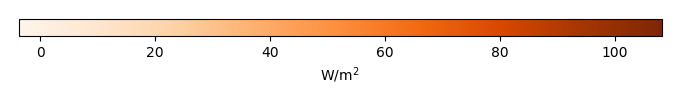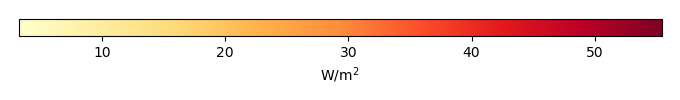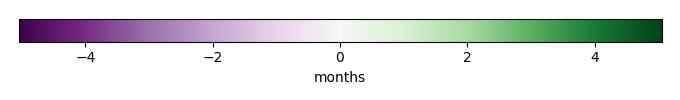# Mean State

Period Mean (original grids) [W/m2]
Model Period Mean (intersection) [W/m2]
Model Period Mean (complement) [W/m2]
Benchmark Period Mean (intersection) [W/m2]
Benchmark Period Mean (complement) [W/m2]
Bias [W/m2]
RMSE [W/m2]
Phase Shift [months]
Bias Score 
RMSE Score 
Seasonal Cycle Score 
Spatial Distribution Score 
Overall Score 
Benchmark [-] 70.6
CLM5PHSOFF [-] 71.0 71.7 63.6 70.7 18.1 0.913 19.1 1.22 0.311 0.118 0.835 0.992 0.475
CLM5PHSON [-] 70.8 71.3 62.2 70.7 18.1 -0.0585 16.6 1.34 0.385 0.166 0.811 0.990 0.504
Period Mean (original grids) [W/m2]
Model Period Mean (intersection) [W/m2]
Model Period Mean (complement) [W/m2]
Benchmark Period Mean (intersection) [W/m2]
Benchmark Period Mean (complement) [W/m2]
Bias [W/m2]
RMSE [W/m2]
Phase Shift [months]
Bias Score 
RMSE Score 
Seasonal Cycle Score 
Spatial Distribution Score 
Overall Score 
Benchmark [-] 47.3
CLM5PHSOFF [-] 46.3 45.9 29.8 47.3 34.2 -1.52 22.4 1.36 0.719 0.468 0.737 0.916 0.662
CLM5PHSON [-] 46.7 46.1 37.1 47.3 34.2 -1.40 22.7 1.43 0.701 0.452 0.726 0.922 0.651
Period Mean (original grids) [W/m2]
Model Period Mean (intersection) [W/m2]
Model Period Mean (complement) [W/m2]
Benchmark Period Mean (intersection) [W/m2]
Benchmark Period Mean (complement) [W/m2]
Bias [W/m2]
RMSE [W/m2]
Phase Shift [months]
Bias Score 
RMSE Score 
Seasonal Cycle Score 
Spatial Distribution Score 
Overall Score 
Benchmark [-] 52.1
CLM5PHSOFF [-] 47.2 46.6 50.5 52.2 23.6 -5.73 21.8 1.05 0.573 0.437 0.862 0.956 0.653
CLM5PHSON [-] 48.3 48.2 51.1 52.2 23.6 -4.01 21.5 1.24 0.578 0.452 0.816 0.972 0.654
Period Mean (original grids) [W/m2]
Model Period Mean (intersection) [W/m2]
Model Period Mean (complement) [W/m2]
Benchmark Period Mean (intersection) [W/m2]
Benchmark Period Mean (complement) [W/m2]
Bias [W/m2]
RMSE [W/m2]
Phase Shift [months]
Bias Score 
RMSE Score 
Seasonal Cycle Score 
Spatial Distribution Score 
Overall Score 
Benchmark [-] 14.9
CLM5PHSOFF [-] 11.9 12.3 9.41 15.0 3.59 -2.61 10.2 0.215 0.806 0.685 1.00 0.534 0.743
CLM5PHSON [-] 15.5 15.7 11.3 15.0 3.59 0.460 12.0 0.219 0.857 0.612 1.00 0.698 0.757
Period Mean (original grids) [W/m2]
Model Period Mean (intersection) [W/m2]
Model Period Mean (complement) [W/m2]
Benchmark Period Mean (intersection) [W/m2]
Benchmark Period Mean (complement) [W/m2]
Bias [W/m2]
RMSE [W/m2]
Phase Shift [months]
Bias Score 
RMSE Score 
Seasonal Cycle Score 
Spatial Distribution Score 
Overall Score 
Benchmark [-] 77.5
CLM5PHSOFF [-] 104. 103. 107. 80.4 34.5 22.7 30.3 2.12 0.206 0.131 0.670 0.415 0.311
CLM5PHSON [-] 95.5 94.9 98.6 80.4 34.5 15.1 25.4 2.16 0.262 0.174 0.660 0.278 0.310
Period Mean (original grids) [W/m2]
Model Period Mean (intersection) [W/m2]
Model Period Mean (complement) [W/m2]
Benchmark Period Mean (intersection) [W/m2]
Benchmark Period Mean (complement) [W/m2]
Bias [W/m2]
RMSE [W/m2]
Phase Shift [months]
Bias Score 
RMSE Score 
Seasonal Cycle Score 
Spatial Distribution Score 
Overall Score 
Benchmark [-] 26.3
CLM5PHSOFF [-] 20.8 20.8 22.8 26.3 3.49 -5.56 10.5 0.122 0.797 0.758 0.993 0.943 0.850
CLM5PHSON [-] 25.1 25.0 25.4 26.3 3.49 -1.27 10.8 0.351 0.884 0.708 0.977 0.990 0.853
Period Mean (original grids) [W/m2]
Model Period Mean (intersection) [W/m2]
Model Period Mean (complement) [W/m2]
Benchmark Period Mean (intersection) [W/m2]
Benchmark Period Mean (complement) [W/m2]
Bias [W/m2]
RMSE [W/m2]
Phase Shift [months]
Bias Score 
RMSE Score 
Seasonal Cycle Score 
Spatial Distribution Score 
Overall Score 
Benchmark [-] 28.0
CLM5PHSOFF [-] 24.6 24.0 32.8 28.1 13.0 -4.18 13.7 0.572 0.643 0.647 0.963 0.871 0.754
CLM5PHSON [-] 26.2 26.5 20.0 28.1 13.0 -1.20 14.1 0.647 0.715 0.610 0.956 0.927 0.763
Period Mean (original grids) [W/m2]
Model Period Mean (intersection) [W/m2]
Model Period Mean (complement) [W/m2]
Benchmark Period Mean (intersection) [W/m2]
Benchmark Period Mean (complement) [W/m2]
Bias [W/m2]
RMSE [W/m2]
Phase Shift [months]
Bias Score 
RMSE Score 
Seasonal Cycle Score 
Spatial Distribution Score 
Overall Score 
Benchmark [-] 57.0
CLM5PHSOFF [-] 56.8 53.8 76.1 57.2 29.3 -3.65 24.8 1.33 0.486 0.402 0.822 0.955 0.613
CLM5PHSON [-] 56.9 56.1 71.3 57.2 29.3 0.854 25.7 1.75 0.570 0.355 0.733 0.960 0.595
Period Mean (original grids) [W/m2]
Model Period Mean (intersection) [W/m2]
Model Period Mean (complement) [W/m2]
Benchmark Period Mean (intersection) [W/m2]
Benchmark Period Mean (complement) [W/m2]
Bias [W/m2]
RMSE [W/m2]
Phase Shift [months]
Bias Score 
RMSE Score 
Seasonal Cycle Score 
Spatial Distribution Score 
Overall Score 
Benchmark [-] 15.7
CLM5PHSOFF [-] 13.9 14.5 8.14 15.8 -6.07 -1.26 11.9 0.151 0.877 0.629 1.00 0.677 0.763
CLM5PHSON [-] 16.7 17.1 9.17 15.8 -6.07 1.09 14.3 0.101 0.921 0.550 1.01 0.753 0.756
Period Mean (original grids) [W/m2]
Model Period Mean (intersection) [W/m2]
Model Period Mean (complement) [W/m2]
Benchmark Period Mean (intersection) [W/m2]
Benchmark Period Mean (complement) [W/m2]
Bias [W/m2]
RMSE [W/m2]
Phase Shift [months]
Bias Score 
RMSE Score 
Seasonal Cycle Score 
Spatial Distribution Score 
Overall Score 
Benchmark [-] 26.8
CLM5PHSOFF [-] 33.5 31.4 49.1 26.9 19.3 4.58 18.7 0.687 0.664 0.367 0.934 0.945 0.656
CLM5PHSON [-] 32.6 31.6 52.1 26.9 19.3 6.35 16.8 0.693 0.667 0.412 0.935 0.916 0.669
Period Mean (original grids) [W/m2]
Model Period Mean (intersection) [W/m2]
Model Period Mean (complement) [W/m2]
Benchmark Period Mean (intersection) [W/m2]
Benchmark Period Mean (complement) [W/m2]
Bias [W/m2]
RMSE [W/m2]
Phase Shift [months]
Bias Score 
RMSE Score 
Seasonal Cycle Score 
Spatial Distribution Score 
Overall Score 
Benchmark [-] 31.2
CLM5PHSOFF [-] 27.3 27.3 27.9 31.7 9.65 -4.50 14.4 0.416 0.692 0.678 0.975 0.585 0.722
CLM5PHSON [-] 33.4 33.4 33.3 31.7 9.65 1.79 15.3 0.325 0.795 0.612 0.982 0.724 0.745
Period Mean (original grids) [W/m2]
Model Period Mean (intersection) [W/m2]
Model Period Mean (complement) [W/m2]
Benchmark Period Mean (intersection) [W/m2]
Benchmark Period Mean (complement) [W/m2]
Bias [W/m2]
RMSE [W/m2]
Phase Shift [months]
Bias Score 
RMSE Score 
Seasonal Cycle Score 
Spatial Distribution Score 
Overall Score 
Benchmark [-] 39.1
CLM5PHSOFF [-] 33.4 38.4 17.5 39.4 16.4 -1.07 16.5 0.811 0.566 0.423 0.866 0.972 0.650
CLM5PHSON [-] 33.9 40.2 7.50 39.4 16.4 1.20 16.5 0.895 0.611 0.424 0.849 0.973 0.656
Period Mean (original grids) [W/m2]
Model Period Mean (intersection) [W/m2]
Model Period Mean (complement) [W/m2]
Benchmark Period Mean (intersection) [W/m2]
Benchmark Period Mean (complement) [W/m2]
Bias [W/m2]
RMSE [W/m2]
Phase Shift [months]
Bias Score 
RMSE Score 
Seasonal Cycle Score 
Spatial Distribution Score 
Overall Score 
Benchmark [-] 8.45
CLM5PHSOFF [-] 7.82 12.3 3.87 8.47 5.81 3.76 10.2 1.15 0.576 0.415 0.925 0.853 0.637
CLM5PHSON [-] 8.46 14.3 3.21 8.47 5.81 6.13 13.3 1.12 0.549 0.258 0.927 0.932 0.585
Period Mean (original grids) [W/m2]
Model Period Mean (intersection) [W/m2]
Model Period Mean (complement) [W/m2]
Benchmark Period Mean (intersection) [W/m2]
Benchmark Period Mean (complement) [W/m2]
Bias [W/m2]
RMSE [W/m2]
Phase Shift [months]
Bias Score 
RMSE Score 
Seasonal Cycle Score 
Spatial Distribution Score 
Overall Score 
Benchmark [-] 14.0
CLM5PHSOFF [-] 13.9 14.7 8.10 14.0 1.27 0.581 11.3 0.239 0.834 0.613 0.994 0.821 0.775
CLM5PHSON [-] 15.9 16.3 7.53 14.0 1.27 1.78 12.9 0.293 0.850 0.544 0.988 0.887 0.763
Period Mean (original grids) [W/m2]
Model Period Mean (intersection) [W/m2]
Model Period Mean (complement) [W/m2]
Benchmark Period Mean (intersection) [W/m2]
Benchmark Period Mean (complement) [W/m2]
Bias [W/m2]
RMSE [W/m2]
Phase Shift [months]
Bias Score 
RMSE Score 
Seasonal Cycle Score 
Spatial Distribution Score 
Overall Score 
Benchmark [-] 50.4
CLM5PHSOFF [-] 60.3 57.9 69.6 50.9 20.2 6.66 23.3 1.01 0.469 0.316 0.866 0.953 0.584
CLM5PHSON [-] 57.0 56.5 63.8 50.9 20.2 8.13 21.5 0.909 0.499 0.361 0.887 0.961 0.614
Period Mean (original grids) [W/m2]
Model Period Mean (intersection) [W/m2]
Model Period Mean (complement) [W/m2]
Benchmark Period Mean (intersection) [W/m2]
Benchmark Period Mean (complement) [W/m2]
Bias [W/m2]
RMSE [W/m2]
Phase Shift [months]
Bias Score 
RMSE Score 
Seasonal Cycle Score 
Spatial Distribution Score 
Overall Score 
Benchmark [-] 49.7
CLM5PHSOFF [-] 45.6 44.5 60.1 49.8 19.0 -5.34 17.0 0.330 0.740 0.704 0.976 0.918 0.808
CLM5PHSON [-] 50.0 49.8 59.9 49.8 19.0 0.529 15.5 0.470 0.831 0.703 0.963 0.847 0.809
Period Mean (original grids) [W/m2]
Model Period Mean (intersection) [W/m2]
Model Period Mean (complement) [W/m2]
Benchmark Period Mean (intersection) [W/m2]
Benchmark Period Mean (complement) [W/m2]
Bias [W/m2]
RMSE [W/m2]
Phase Shift [months]
Bias Score 
RMSE Score 
Seasonal Cycle Score 
Spatial Distribution Score 
Overall Score 
Benchmark [-] 25.2
CLM5PHSOFF [-] 25.5 25.4 30.4 25.4 10.7 -0.0146 11.2 0.145 0.800 0.702 0.992 0.933 0.826
CLM5PHSON [-] 30.4 30.4 34.3 25.4 10.7 4.98 13.7 0.267 0.814 0.625 0.984 0.921 0.794
Period Mean (original grids) [W/m2]
Model Period Mean (intersection) [W/m2]
Model Period Mean (complement) [W/m2]
Benchmark Period Mean (intersection) [W/m2]
Benchmark Period Mean (complement) [W/m2]
Bias [W/m2]
RMSE [W/m2]
Phase Shift [months]
Bias Score 
RMSE Score 
Seasonal Cycle Score 
Spatial Distribution Score 
Overall Score 
Benchmark [-] 27.2
CLM5PHSOFF [-] 22.9 23.3 31.4 27.2 14.3 -3.83 12.5 0.648 0.680 0.590 0.951 0.953 0.753
CLM5PHSON [-] 25.9 26.4 31.7 27.2 14.3 -0.645 13.1 0.668 0.729 0.542 0.946 0.978 0.748
Period Mean (original grids) [W/m2]
Model Period Mean (intersection) [W/m2]
Model Period Mean (complement) [W/m2]
Benchmark Period Mean (intersection) [W/m2]
Benchmark Period Mean (complement) [W/m2]
Bias [W/m2]
RMSE [W/m2]
Phase Shift [months]
Bias Score 
RMSE Score 
Seasonal Cycle Score 
Spatial Distribution Score 
Overall Score 
Benchmark [-] 90.0
CLM5PHSOFF [-] 93.2 93.3 93.4 90.2 27.7 3.06 18.7 1.80 0.408 0.134 0.743 0.993 0.482
CLM5PHSON [-] 91.4 91.5 87.6 90.2 27.7 1.06 14.5 1.90 0.445 0.203 0.727 0.920 0.499
Period Mean (original grids) [W/m2]
Model Period Mean (intersection) [W/m2]
Model Period Mean (complement) [W/m2]
Benchmark Period Mean (intersection) [W/m2]
Benchmark Period Mean (complement) [W/m2]
Bias [W/m2]
RMSE [W/m2]
Phase Shift [months]
Bias Score 
RMSE Score 
Seasonal Cycle Score 
Spatial Distribution Score 
Overall Score 
Benchmark [-] 69.7
CLM5PHSOFF [-] 62.3 61.7 66.9 69.8 17.5 -7.97 22.6 0.967 0.594 0.435 0.900 0.988 0.671
CLM5PHSON [-] 65.2 64.8 69.8 69.8 17.5 -4.76 20.5 0.946 0.636 0.469 0.905 0.990 0.694
Period Mean (original grids) [W/m2]
Model Period Mean (intersection) [W/m2]
Model Period Mean (complement) [W/m2]
Benchmark Period Mean (intersection) [W/m2]
Benchmark Period Mean (complement) [W/m2]
Bias [W/m2]
RMSE [W/m2]
Phase Shift [months]
Bias Score 
RMSE Score 
Seasonal Cycle Score 
Spatial Distribution Score 
Overall Score 
Benchmark [-] 22.7
CLM5PHSOFF [-] 23.3 24.4 14.3 23.0 8.02 1.50 12.7 0.870 0.515 0.458 0.912 0.947 0.658
CLM5PHSON [-] 27.6 27.9 17.3 23.0 8.02 4.79 15.2 0.929 0.510 0.376 0.917 0.948 0.625

# Temporally integrated period mean

BENCHMARK MEANMODEL MEANBIASBIAS SCORERMSERMSE SCOREBENCHMARK MAX MONTHMODEL MAX MONTHDIFFERENCE IN MAX MONTHSEASONAL CYCLE SCORESPATIAL TAYLOR DIAGRAMMODEL COLORS# Spatially integrated regional mean

MODEL COLORSREGIONAL MEANANNUAL CYCLEMONTHLY ANOMALYANNUAL CYCLE# All Models

BenchmarkCLM5PHSOFFCLM5PHSON# Data Information

creation_date: Thu Dec 5 00:02:00 PST 2013

Conventions: Please contact Prof. James Randerson (Email: jranders@uci.edu) or Dr. Mingquan Mu (mmu@uci.edu) for any question

source_file: This product is generated from monthly FluxNet MTE observations

title: derived FluxNet MTE in 0.5x0.5

Approach: I read this variable from data file, and then save the data in NetCDF format by each month and each year. Meanwhile, I also converted the unit from g C m-2 day-1 to Kg C m-2 s-1.

Temporal resolution: monthly

General information: This product was derived from Fluxnet-MTE originally from Monthly estimates of global biosphere-atmosphere fluxes from Biogeochemistry group at Max Planck Institute in Jena, Germany.

Spatial resolution: 0.5x0.5 degree